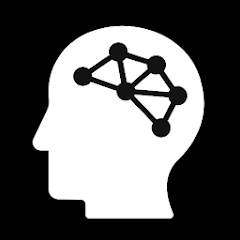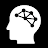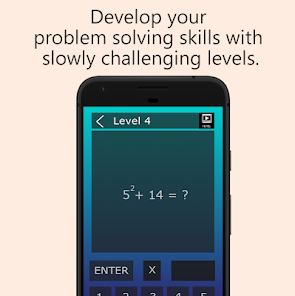# The Math10+EveryoneWe all require some numerical skills in our lives, whether it is to calculate our weekly shopping bill or to budget how to use our monthly income. Flexibility of thought and lateral thinking processes are a few skills which are needed in order to solve these problems. Mathematical intelligence generally represents your ability to reason and to calculate basic arithmetic computations. It also helps you to understand geometric shapes and manipulate equations.

Mathematical intelligence is a strong indicator of general intelligence because many every day mental tasks require arithmetical operations even though numbers may not be involved.
Since we saw those reasons to make a mathematical game that you can make calculations of arithmatical problems while spending tasteful hours.
Updated on
Dec 20, 2018

## Data safety